## Saturday, June 18, 2011

### Area of a Triangle Given Coordinates

There are at least 6 methods to determine the area of a triangle given the coordinates of the vertices.  Some of the methods apply to triangles only, and some are more generic.  We’ll find two clear winners for this problem (that are nearly the same), but also notice, that if we were given different information (such as lengths of sides instead of coordinates of vertices) it would change the winner.  I am focusing here on 2D coordinates.  Here's a picture of our triangle:

Heron’s Formula

Heron’s formula uses the lengths a, b, and c of the sides to determine the area of the triangle: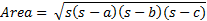where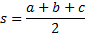To use this formula, we must determine the lengths of the sides.  We use the Pythagorean theorem to do so: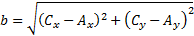This whole calculation involves 4 square roots, 9 multiplications, 1 division by 2, and 14 addition/subtractions.  This method is very expensive compared with the other methods.  However, if we were given the lengths of the sides and not the coordinates of the vertices, it would be less expensive than the alternative.  The alternative would require the use of trigonometric functions (law of cosines and law of sines) in order to determine the height to use the formula A = bh/2.  Trig functions are way more expensive (time-wise) than square roots.

Note that this method could be extended to work given 3D coordinates, since the Pythagorean theorem extends to 3D coordinates.  It would be a bit more expensive in 3D.

Coordinates Method

This method can be used with any polygon – it need not be a regular polygon (all sides and interior angles equal) or a triangle.  The only requirement is that none of the sides intersect.  The method is performed as follows: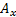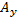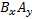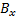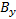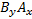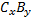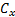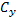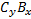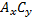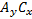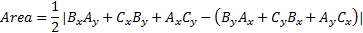This method requires 6 multiplications, 1 division by 2, and 5 addition/subtractions – easily outperforming Heron’s formula when we’re given the coordinates.

Double Meridian Distance (DMD)

This is another generic method that applies in the same circumstances as the coordinates method.  It is always more efficient than using the method of coordinates.  In a spreadsheet is would look like this:

 A B C D E F 1 x-coord y-coord delta y delta x DMD 2*A 2 Ax Ay =B3-B2 =A3-A2 =D2 =E2*C2 3 Bx By =B4-B3 =A4-A3 =E2+D2+D3 =E3*C3 4 Cy Cy =B5-B4 =A5-A4 =E3+D3+D4 =E4*C4 5 Ax Ay 6 =sum(F2:F4)/2

This method requires 3 multiplications, 1 division by 2, and 12 addition/subtractions.  Although we have way more addition subtraction, we have half the number of multiplications.  Since multiplication and division are way more expensive than addition and subtraction, this method is computationally superior to the method of coordinates.

The Usual Method (sorta)

Youre probably wondering why I havent just taken half the base times the height.  Okay wise guy, where`s the base?  How about the height?  Can we calculate them?  Yes, but are you sure you know how much work you’re in for?  You say, isn’t the area just

No, it’s not that simple.  The base must be the length of one of the sides.  The height must be measured perpendicularly from the chosen base to the vertex which is not on the base.  But we can find the area using this method if we follow it through to the bitter end.  Let’s do it.

First, we calculate the length of the base, which we will choose as side b above:Now, we need to calculate the height, h, between point B and line AC (side b).  This will be nontrivial and slightly painful.

We determine the equation of the line through A and C using the point-slope form: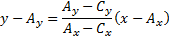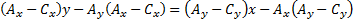We re-express this result as

where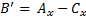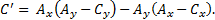At this point we use a formula for the distance between a point and a line that uses the equation of the line as expressed above:

Finally, we get to say “Area = bh/2,” and I hope you are satisfied!  We needed 2 square roots, 9 multiplication/divisions, and 11 addition/subtractions.  This method gives you the right answer, but there’s got to be a better way!

A Better Way

Let’s redraw our triangle with a fancy rectangular border:
Okay, it’s not that fancy, but it’s useful!  First, let’s calculate L, R, λ, and ρ.We can calculate the area of our triangle now:I’ve skipped showing all of the simple expansion and simplification steps above because I’m getting tired of all that typing.  But here’s the vital statistics: 2 multiplications, 1 division by 2, and 5 addition/subtractions.

Cross Product Method

This method not only works in 3D, it requires 3D – sorta.  We’ll apply it to the 2D case here – we just augment our coordinates with zeros for the z-coordinates.  It goes like this: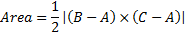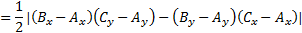The final score for this method is 2 multiplications, 1 division by 2, and 5 addition subtractions.  This method is the clear winner since it is tied with the previous method in 2D but is easily applied to 3D with an increase in cost.

Method Comparison Table
 method square roots multiplication/division division by 2 addition/ subtraction Heron’s Formula 4 9 1 14 Coordinates Method 0 6 1 5 DMDs 0 3 1 12 “Usual” Method 2 9 1 11 A Better Way 0 2 1 5 Cross Product 0 2 1 5

* Note:  I've put division by 2 in a separate category because division and multiplication by 2 can be implemented more efficiently on the computer than general case multiplication and division.